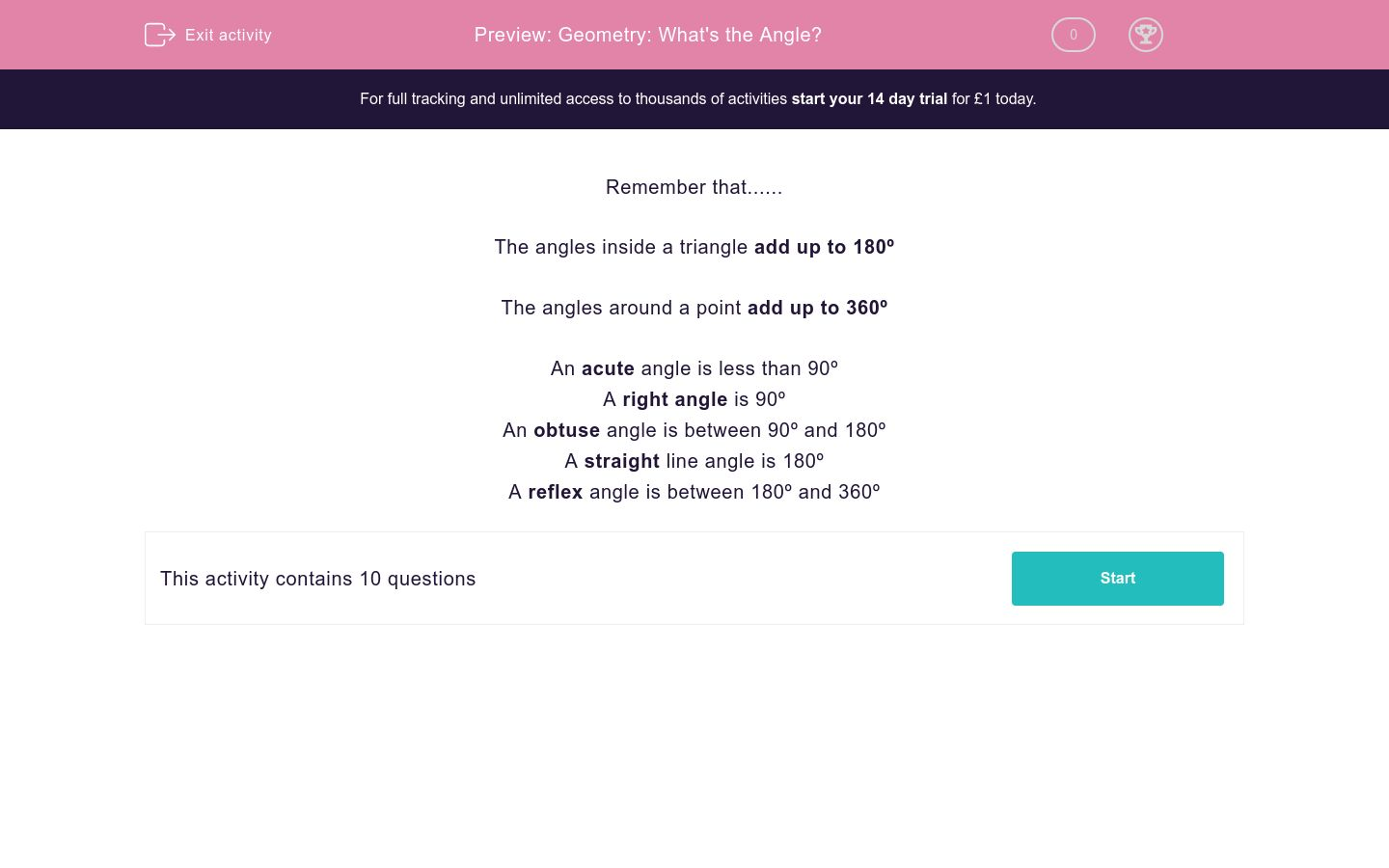# Geometry: What's the Angle?

In this worksheet, students answer a variety of angle-based questions.Key stage:  KS 2

Curriculum topic:   Maths and Numerical Reasoning

Curriculum subtopic:   2D Shapes: Circles, Angles and Bearings

Difficulty level:### QUESTION 1 of 10

Remember that......

The angles inside a triangle add up to 180º

The angles around a point add up to 360º

An acute angle is less than 90º

A right angle is 90º

An obtuse angle is between 90º and 180º

A straight line angle is 180º

A reflex angle is between 180º and 360º

---- OR ----